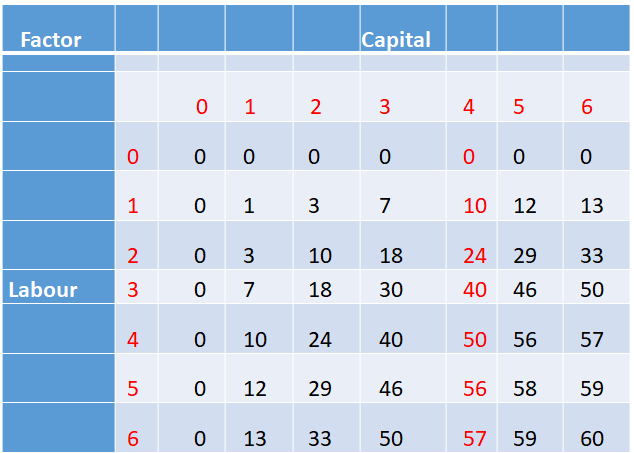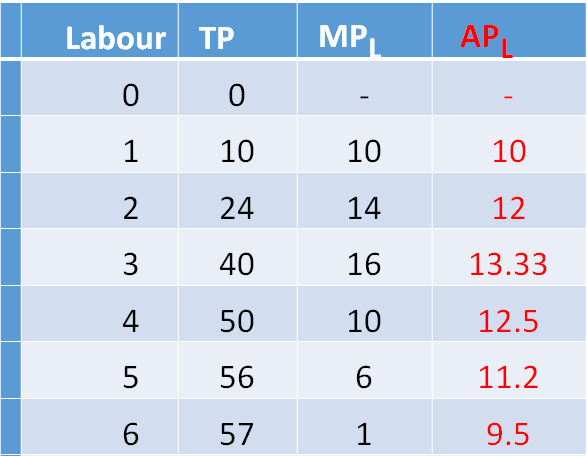Economics (NCERT) Notes

3.4 Total Product, Average Product, and Marginal Product

Total Product•If we vary a single input and keep all other inputs constant, we get different levels of output for different levels of that input.
•This relationship between the variable input and output, keeping all other inputs constant, is often referred to as Total Product (TP) of the variable input.
•The total product of labour schedule with K2 = 4 is shown in Table.
•This is also sometimes called total return to or total physical product of the variable input.

Calculation of Total Product

Average Product
•Average product is defined as the output per unit of variable input.
•Average Product is calculated as APL=TPL/L

Calculation of Average Product•The last column of table gives us a numerical example of average product of labour (with capital fixed at 4) for the production function described in table.
•Values in this column are obtained by dividing TP by L

Marginal Product
•Marginal product of an input is defined as the change in output per unit of change in the input when all other inputs are held constant.
•When capital is held constant, the marginal product of labour is
•MPL =Change in Output/Change in Input =∆TPL/ ∆ L
•where represents the change of the variable.

Calculation of Marginal Product

•The third column of table 3.2 gives us a numerical example of Marginal Product of labour (with capital fixed at 4) for the production function described
•Values in this column are obtained by dividing change in TP by change in L.
•MPL =∆TPL/ ∆ L

Attributes of Marginal Product
•Since inputs cannot take negative values, marginal product is undefined at zero level of input employment.
•For any level of an input, the sum of marginal products of every preceding unit of that input gives the total product.
•Hence, the total product is the sum of marginal products.

Average and marginal products•Average product of an input at any level of employment is the average of all marginal products up to that level.
•Average and marginal products are often referred to as average and marginal returns, respectively, to the variable input.

 Related Articles
 • 6.8 Behaviour of Firms in Oligopoly • 6.1 Non-competitive Markets • 5.8 Applications of Supply-Demand Analysis • 5.6 Market Equilibrium: Free Entry and Exit • 5.5 Impact of Shift in Supply and Demand • 5.2 Market Equilibrium for Fixed Number of Firms • 5.1 Market Equilibrium • 4.10 Price Elasticity of Supply • 4.9 Market supply Curve • 8.1 Importance of Infrastructure
 Recent Articles
 • Innovation and Creativity • Love and hatred • Religion and Spirituality • Tulsidas • Bureaucrat at the Temple • Getting Fooled for Kindness • Burning the boat • Three Masons • Two Salesmen • Bhasmasur